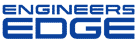# True position calculation

[ Home ][ Forum Archive ] [ Active Engineering Forum ]

Posted By<" ">Terri O'Day on May 23, 2002 at 14:43:07:

Can someone explain why their is a factor of 2 in the calculation for true position ?
2 *SqRT(delta X ^2)+ (delta y^2).

< "> Subject: Re: True position calculation